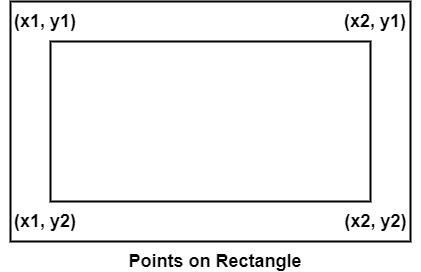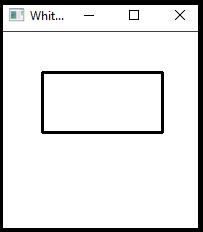# How to draw a rectangle in OpenCV using C++?

To draw a rectangle, we need four points. Look at the following figure.In the figure, there are four points x1, x2, y1 and y2. These four points are forming the four coordinates. To draw a rectangle using OpenCV, we have to define these points and show the rectangle we need a matrix. We have to declare other relevant values like the color of the line and line width.

The basic syntax of this method is as follows −

## Syntax

rectangle(whiteMatrix, starting, ending, line_Color, thickness);

The following program represents how to draw a rectangle in OpenCV.

## Example

#include<iostream>
#include<opencv2/highgui/highgui.hpp>
#include<opencv2/imgproc/imgproc.hpp>
using namespace cv;
using namespace std;
int main() {
Mat whiteMatrix(200, 200, CV_8UC3, Scalar(255, 255, 255));// Declaring a white matrix//
Point starting(40, 40);//Declaring the starting coordinate//
Point ending(160, 100);//Declaring the ending coordinate
Scalar line_Color(0, 0, 0);//Color of the rectangle//
int thickness = 2;//thickens of the line//
namedWindow("whiteMatrix");//Declaring a window to show the rectangle//
rectangle(whiteMatrix, starting, ending, line_Color, thickness);//Drawing the rectangle//
imshow("WhiteMatrix", whiteMatrix);//Showing the rectangle//
waitKey(0);//Waiting for Keystroke
return 0;
}

## Output